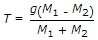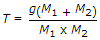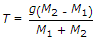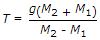# Civil Engineering - Applied Mechanics

1.
If two bodies of masses M1 and M2(M1 > M2) are connected by alight inextensible string passing over a smooth pulley, the tension in the string, will be given byExplanation:
No answer description is available. Let's discuss.

2.
For a simple pendulum, the period of one oscillation is
l/2g
2g/l
l/g
g/2l.
Explanation:
No answer description is available. Let's discuss.

3.
A load of 500 kg was lifted through a distance of 13 cm. by an effort of 25 kg which moved through a distance of 650 cm. The velocity ratio of the lifting machine is
50
55
60
65
70.
Explanation:
No answer description is available. Let's discuss.

4.
The angle of friction is :
The ratio of the friction and the normal reaction
The force of friction when the body is in motion
The angle between the normal reaction and the resultant of normal raction and limiting friction
The force of friction at which the body is just about to move.